# College Chemistry : Nomenclature and Functional Groups

## Example Questions

### Example Question #1 : Nomenclature And Functional Groups

Name the compound: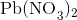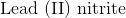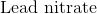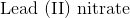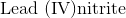Explanation:

First, recognize that this is an ionic compound.

Identify the cation and the anion. The cation is lead, and the anion is nitrate.

Since lead can form more than one type of ion, figure out the charge associated with the given cation.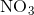has a charge of. Since there arenitrate ions, the charge for lead must be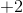. Remember to put the charge of the cation in parentheses when naming the compound.

The name of this compound is then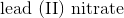.

### Example Question #2 : Nomenclature And Functional Groups

What is the correct name for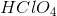?

Chlorous acid

Hydrochloric acid

Perchloric acid

None of these

Chloric acid

Perchloric acid

Explanation:consists of a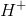ion bonded to a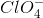ion, which is a perchlorate ion. Thus, the name of the ion's corresponding acid is perchloric acid.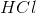is the molecular formula for hydrochloric acid,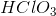is the formula for chloric acid, while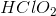is the formula for chlorous acid.

### Example Question #3 : Nomenclature And Functional Groups

Consider the following the trend of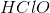,,, and. Identify the name of the ternary acid,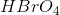.

Perbromic acid

Hypobromic acid

Hydorbromic acid

Bromous acid

Hypobromous acid

Perbromic acid

Explanation:

Because there are four oxygen atoms like in perchloric acid, the correct name is perbromic acid. Hypobromic acid is not a valid name for an acid since the "hypo-" prefix refers to one or two oxygen atoms but the "-ic" suffix refers to either three or four oxygen atoms. Hypobromous and bromous acid refer to one and two oxygen atoms, respectively. Hydrobromic acid has no oxygen atoms.

### Example Question #4 : Nomenclature And Functional Groups

What is the correct nomenclature for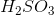?

Hydrogen sulfate

Hydrogen sulfide

Sulfurous acid

Sulfuric acid

Hydrogen sulfite

Sulfurous acid

Explanation:

The correct nomenclature foris sulfurous acid. Oxyanions ending in -ate form oxyacids ending in -ic. For example, sulfate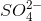becomes sulfuric acid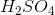. Oxyanions ending in -ite form oxyacids ending in -ous. In this problem, sulfite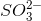becomes sulfurous acid.

### Example Question #5 : Nomenclature And Functional Groups

What is the correct molecular formula for cobalt (II) sulfite?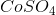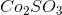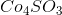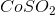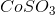Explanation:

The correct molecular formula for cobalt (II) sulfite is. The charge on cobalt (II) is. When combined with sulfite, the positive and negative charges cancel out to create a neutral compound.

### Example Question #31 : Compounds And Molecules

What is the oxidation state of manganese (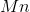) in the polyatomic permanganate anion (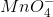)?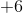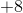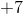Explanation:

When assigning oxidation states to elements of a given compound, non-transition metal elements are assigned specific oxidation states corresponding to their group number and valence relative to a complete octet.

Group 1 elements have 1 valence electron and an oxidation state of +1.

Group 2 elements have 2 valence electrons and an oxidation state of +2.

Group 8 elements (the noble gases) have a complete octet, thus their assigned oxidation state is 0.

Group 7 elements (halogens) have 7 valence electrons and an oxidation state of -1.

Group 6 elements such as oxygen have 6 valence electrons and an oxidation state of -2.

Permanganate has 4 oxygen atoms and an overall charge of -1. The oxidation state of theatom may be found by first calculating the combined oxidation state of the oxygen atoms: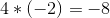and finding the difference between their combined oxidation state and the overall charge of the ion (-1):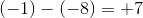### Example Question #32 : Compounds And Molecules

According to VSEPR theory, for the ammonium ion (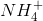), the electron-pair geometry is __________ and the molecular geometry is __________.

tetrahedral . . . trigonal pyramidal

trigonal pyramidal . . . tetrahedral

tetrahedral . . . tetrahedral

trigonal pyramidal . . . trigonal pyramidal

tetrahedral . . . tetrahedral

Explanation:

Both the electron-pair and molecular geometries of ammonium are tetrahedral, as opposed to ammonia (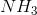), in which the electron-pair geometry is tetrahedral, but the molecular geometry is trigonal pyramidal. As evidenced below:

Ammonia: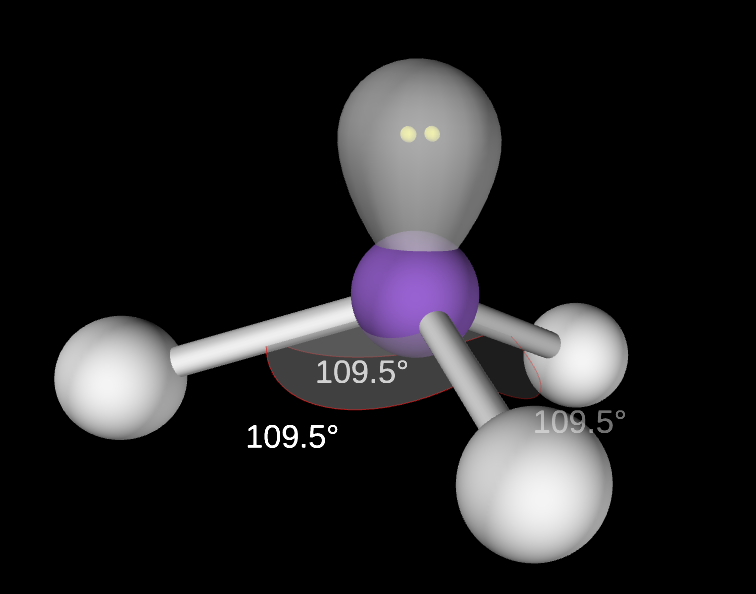Note that the difference in geometry arises in the presence of the lone pair on nitrogen in ammonia. The lone pair only contributes to molecular geometry, but does not contribute to electron-pair geometry. Bonding electrons, however, contribute to both electron and molecular geometries.

Ammonium: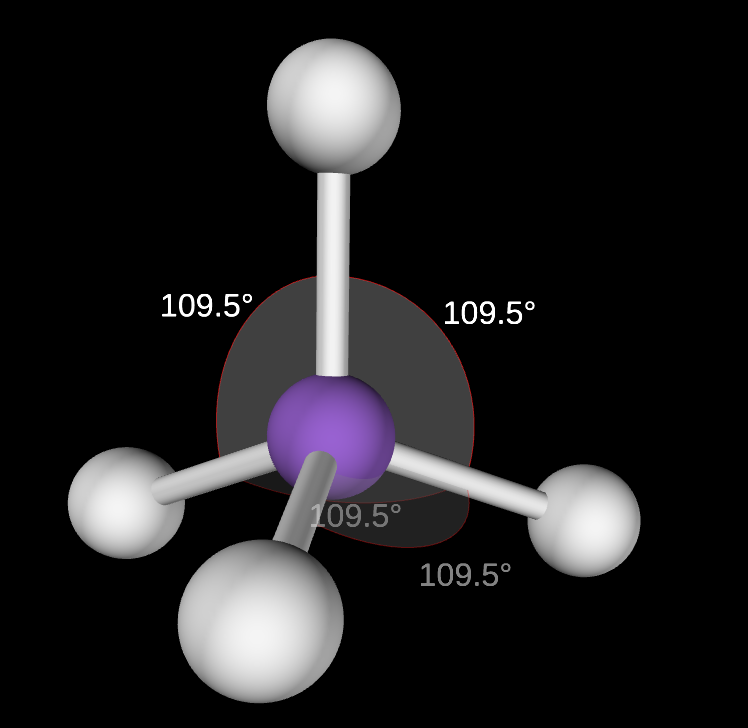In ammonium, the lone pair seen in ammonia is shared in a bond with an additional hydrogen atom.

### Example Question #33 : Compounds And Molecules

What are the mass percents of C, H, and O, respectively, in a molecule of glucose monomer (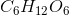)?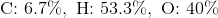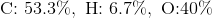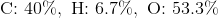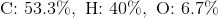Explanation:

On average, the molar masses of carbon, hydrogen, and oxygen are approximately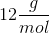,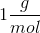, and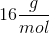, respectively.

The molar mass of a molecule of glucose is: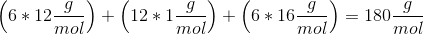Each term added in the previous step is the mass of each element present in a mole of glucose, so the mass percent of its components are found by dividing their respective contributions to the molar mass by the molar mass: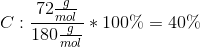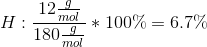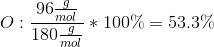### Example Question #41 : Compounds And Molecules

What is the name for the following polyatomic ion: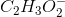(also written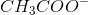)?

Carbon trihydrogen monocarbon dioxide

Acetate

Dicarbon trihydrogen dioxide

Dichromate

Oxalate

Acetate

Explanation:(also written as) is the formula for the acetate ion. Most polyatomic ions tend to follow certain naming trends, but acetate is one of the ions that does not follow those naming trends, so memorize this one since it shows up frequently in chemistry.

### Example Question #6 : Nomenclature And Functional Groups

Name the compound: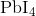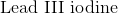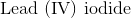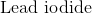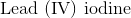Explanation:

Start by identifying the cation and the anion of this ionic compound.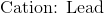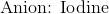Next, deduce the charge found on the lead. Since we know that ion for iodine normally has acharge, we can figure out that the lead must have a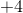charge in order to create the neutral compound.

Next, remember that the anion name of iodine is iodide.

Finally, put the name together by using the following formula: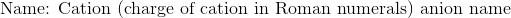Thus, the name of the compound is.